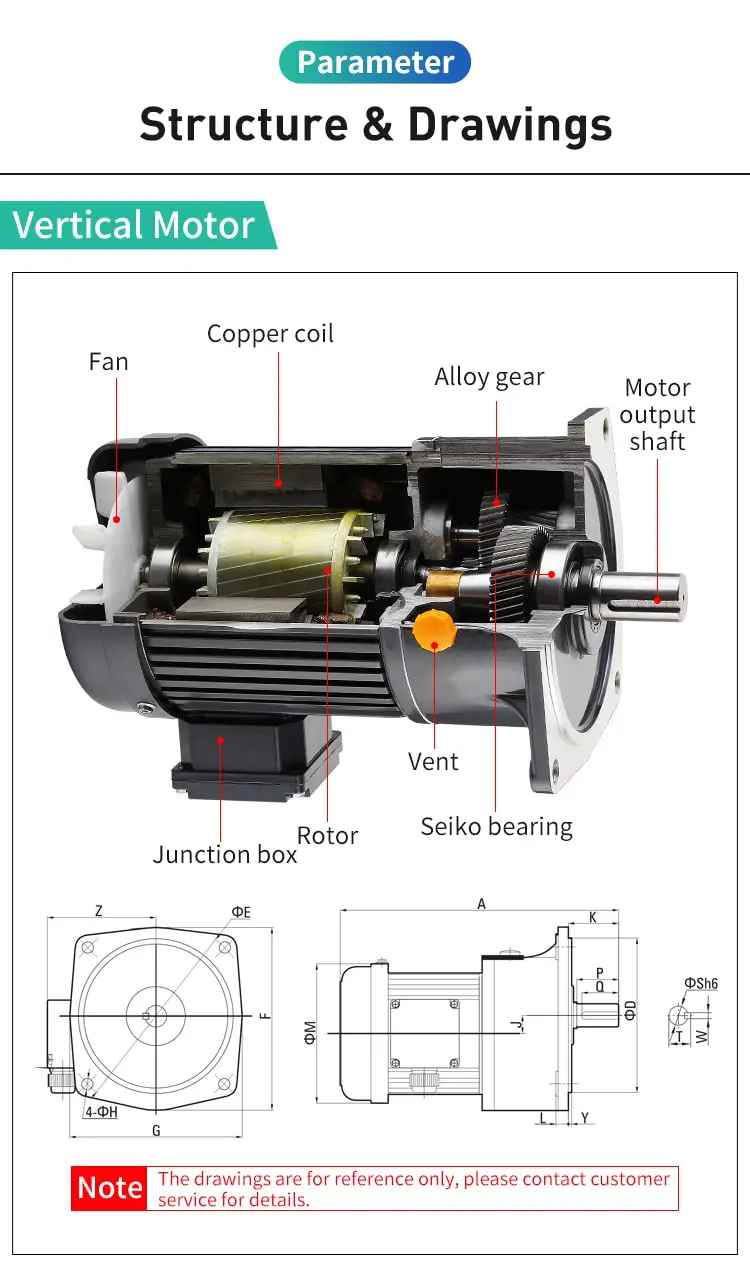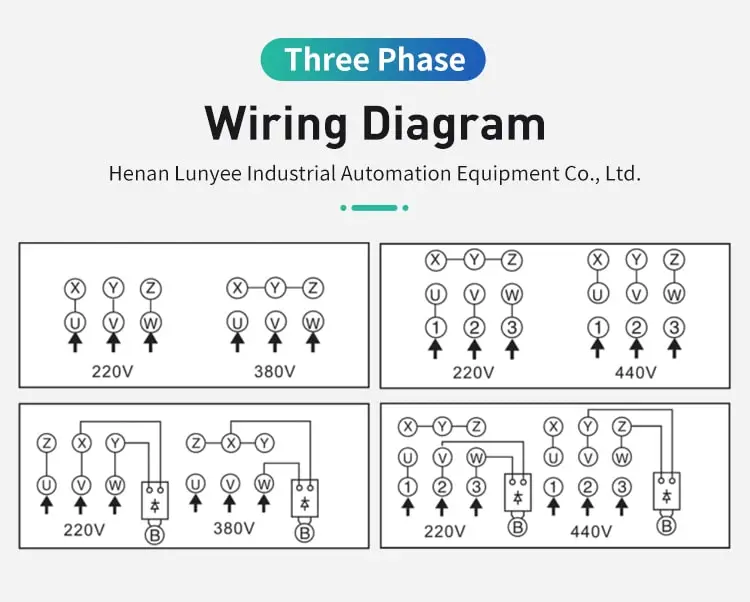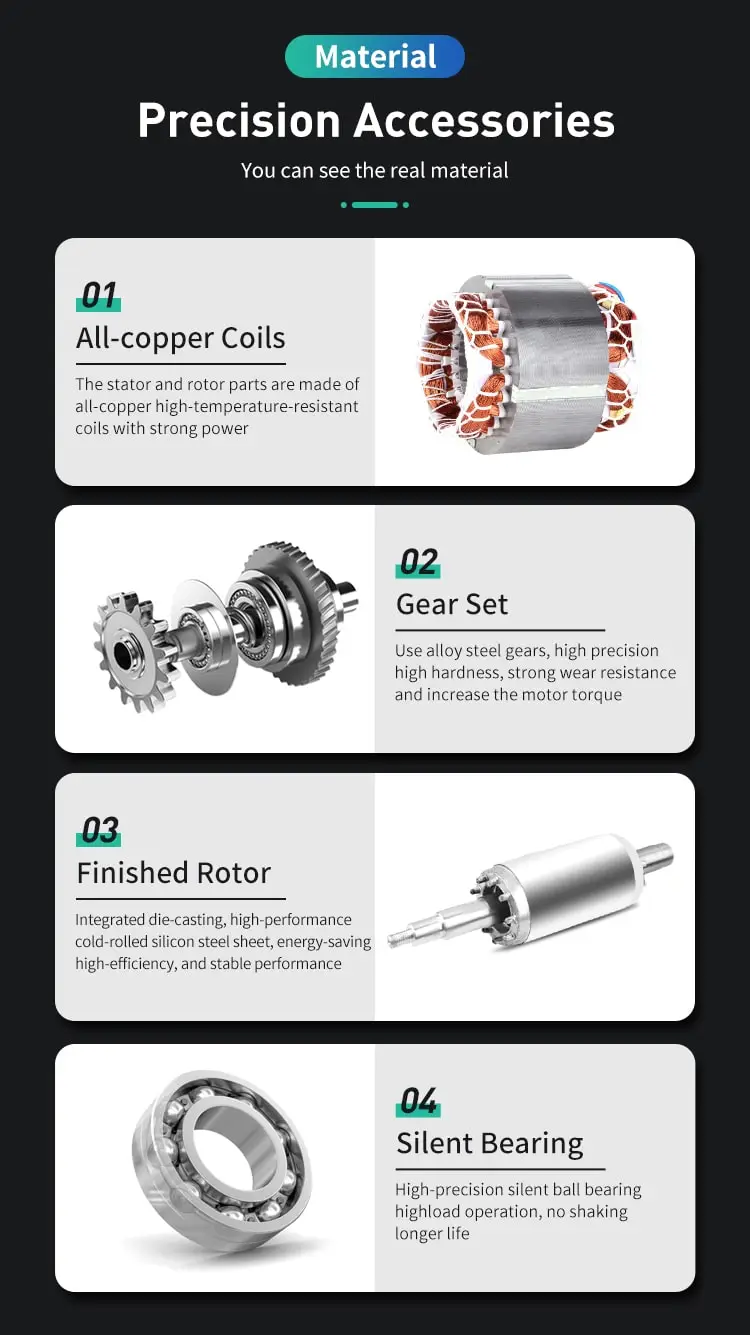By Jasmine 2023-02-28 11:50:16

#### How to calculate slip in induction motor?

The slip in an induction motor is calculated using the formula: Slip = (Ns - Nr) / Ns x 100%, where Ns is the synchronous speed and Nr is the rotor speed. Synchronous speed can be calculated using the formula Ns = (120 x f) / p, where f is the supply frequency and p is the number of poles. Slip values typically range from 1% to 5%.

The slip of an induction motor is a measure of the difference between the speed of the stator's rotating magnetic field and the speed of the rotor. It is an important parameter in the operation of an induction motor, as it affects the motor's torque, efficiency, and power output.

To calculate the slip of an induction motor, it is helpful to consider the basic operating principle of this type of motor. An induction motor uses electromagnetic induction to generate rotational motion, which is accomplished by creating a magnetic field around a coil of wire.

In an induction motor, the magnetic field is produced by the stator, which is a stationary component that consists of a series of copper or aluminum coils. The stator is typically mounted on the outer shell of the motor, and it is connected to the electrical power supply. When an electrical current flows through the stator coils, it creates a magnetic field that extends into the rotor, which is a moving component that is mounted on the motor's shaft.

The rotor is typically made up of a series of conductive bars that are connected to a series of short-circuited end rings. When the rotor is placed in the magnetic field produced by the stator, it experiences a force known as the "rotor reaction," which causes it to rotate. This rotational motion is transferred to the motor's shaft, which can then be used to drive a load.

The key to the operation of an induction motor is the interaction between the magnetic fields of the stator and rotor. When the stator's magnetic field rotates, it creates a current in the rotor's conductive bars, which in turn creates its own magnetic field. This magnetic field interacts with the stator's magnetic field, resulting in a force that causes the rotor to rotate.

The slip of an induction motor is the difference between the speed of the stator's magnetic field and the speed of the rotor. It is typically expressed as a percentage, and it is calculated using the following formula:

Slip = (S - N) / S

Where:

S = the speed of the stator's magnetic field

N = the speed of the rotor

For example, if the speed of the stator's magnetic field is 1,000 RPM, and the speed of the rotor is 950 RPM, the slip of the induction motor would be calculated as follows:

Slip = (1,000 - 950) / 1,000 = 0.05 or 5%

In general, the slip of an induction motor is a function of the load on the motor. As the load on the motor increases, the slip of the motor also increases, which reduces the motor's efficiency and power output. To maintain the motor's efficiency and power output, it is important to control the slip of the motor by adjusting the load or the speed of the stator's magnetic field.

Overall, the slip of an induction motor is an important parameter in the operation of this type of motor. It is calculated as the difference between the speed of the stator's magnetic field and the speed of the rotor, and it is a function of the load on the motor. By controlling the slip of the motor, it is possible to maintain the motor's efficiency and power output.